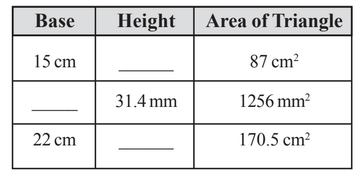# Q. 4.     Find the missing values:R Riya

We know that

Area of triangle

a) Here, the base and area of the triangle is given

b) Here height and area of the triangle is given

c) Here base and area of the triangle is given

Exams
Articles
Questions